# Sensors - Optical Time-of-Flight Ranging - EdsCave

Go to content

## Sensors - Optical Time-of-Flight Ranging

Sensors

24 Jan 2016

Optical Time-of-Flight Ranging
is a technology that has been around for a while, but with recent advances in silicon integration is starting to become inexpensive enough to find many new uses.

The basic idea behind time-of-flight (TOF) ranging is pretty simple: you send out a short pulse of light from a LASER, LED or similar device, receive the reflection using a photodiode or other fast optical detector, and measure the time elapsed between transmission and receipt (Figure 1). This delay time is proportional to the distance between the TOF system and the reflective target (Figure 2).  From a conceptual standpoint this is not very different than how RADAR works, except that the wavelength of light (< 1 um)  is much, much smaller than that of the microwaves employed by radar systems (1mm-1cm).

Figure 1 - Conceptual Optical Time-of-flight Measurement System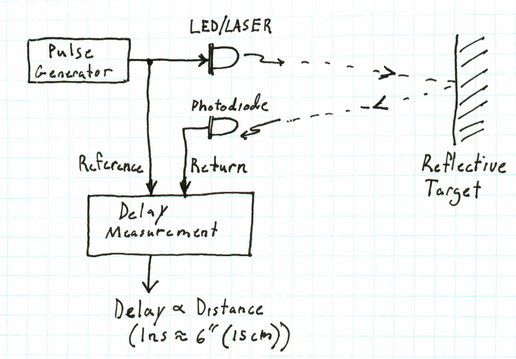Figure 2 - Signals for Conceptual System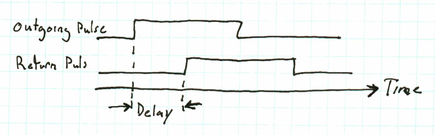Although simple in concept, there are a number of non-trivial technical challenges that must be overcome to realize a practical TOF ranging system:

• Generation of short, high-intensity optical pulses with fast edges (rise and fall times).

• Fast-response photodetectors and amplification and discrimination electronics.

• Accurate measurement of short time intervals.

Although all of the above topics are interesting, this blog article will focus on the last one - measurement of short time intervals.

In the context of an optical TOF ranging system, a 'short' interval can mean anything between a few tens of picoseconds and a few microseconds. This is because light and other electromagnetic radiation travels very quickly compared to any commonly encountered physical object. For example, in one microsecond, a beam of light travels 300m (~1000 ft). In comparison, a sniper bullet moving at 1000m/s moves less than 1mm (1/25th of an inch) in that microsecond.  From a precision standpoint, being able to resolve time to the nearest microsecond would only let you resolve range in an optical TOF system to within 150m (500 feet).  While there are probably ranging applications where this much resolution is fine, there are many more in which it is essential to resolve distance down to the meter, centimeter, or even millimeter range.

First, let's consider a simple 'brute-force' method of time measurement - a counter-based digital timer (Figures 3).  In this scheme, you have a fast reference clock, which you enable or gate (turn-on) when you launch the optical pulse, and then turn off when the return pulse is detected.  The elapsed time is recorded as the final count in the counter when the clock is disabled (Figure 4).

Figure 3 - Measuring Time with a Fast Counter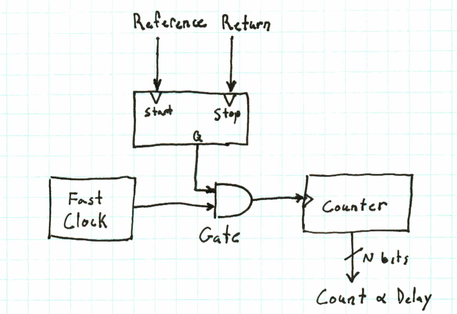Figure 4 - Signals for Counter-based Measurement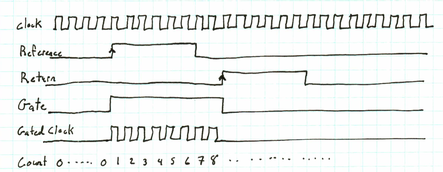While a counter-based time measurement is conceptually simple, easy to implement, and in pretty common use (my bench frequency counter has a 'gate' input that supports this kind of function), it suffers from a serious limitation. The time resolution is limited by the frequency of the 'fast' clock.  While the reference clock for my bench counter is 10MHz (which only provides 100ns of time resolution) clock references up to 500MHz or so are readily available, and not even all that expensive.  500 MHz, however, only provides 2ns of time resolution - which translates into 0.3m (1 foot) of ranging resolution. This might be fine for many applications, particularly those measuring ranges over distances measured in kilometers.

For some applications, however, such as measuring the distance to the moon (~400,000 km away),   'meter-scale' accuracy is simply not good enough.  Even for more mundane applications more accuracy may be required. Fortunately there are many ways of measuring time delays that provide more resolution than a counter-based timer.

If one has a way of generating a precise and known delay, it is possible to measure an unknown delay by comparison.  Consider the block-diagram shown in Figure 5. In this system, the reference pulse is fed through a programmable delay. Although there are many ways to realize a programmable delay - some analog, some digital - digital versions of this function are readily available. One such implementation is the MC100EP196 ECL Programmable Delay.  This device allows you to insert a delay into a digital signal path up to about 10ns, with a programmable resolution of ~10 picoseconds. In 10 picoseconds, light travels about 3mm (1/8").

Figure 5 - Measuring Delays by Comparison with Known Delay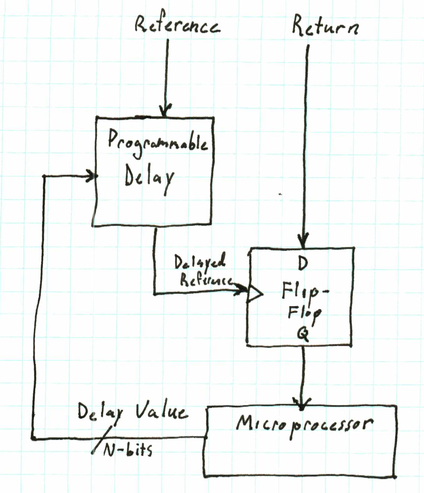The way in which this technique is used is to compare the arrival time of the delayed reference signal to that of the detected return signal. While there are many ways to do this, one particular simple method is to use the delayed reference to clock a 'D' flip-flop and feed the return signal into the D-input. If the return signal goes HIGH (arrives) before the delayed reference goes HIGH, the flip-flop's output latches HIGH. If the return signal arrives after delayed reference goes HIGH, the flip-flop's output latches a LOW (Figure 6). By dynamically adjusting the delay so that it is just at the point where the return and delayed reference arrive at the flip-flop at the same time, one can determine the time by which the return signal is delayed.  Making this adjustment requires monitoring the flip-flop's output over multiple pulses and making appropriate adjustments to the delay - hence the need for a microcontroller or something else to provide some algorithmic intelligence. Several years ago I had the opportunity to try this approach in a non-TOF context - measuring clock skew for a semiconductor product I worked on.

Figure 6 - Signals for Delay-comparison Method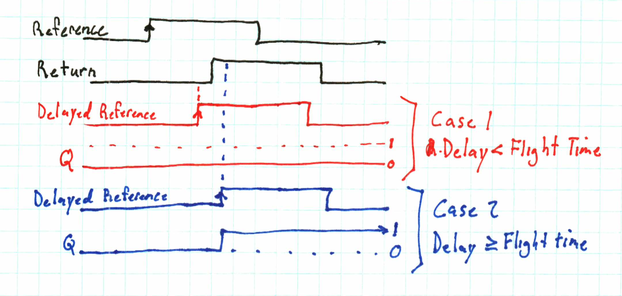The two previous delay-measurement techniques were very digital. Another set of approaches involves converting time into an analog measurement.  One of the simplest ways of doing this is to perform a phase comparison of the reference and return signals, and then filter the output into a DC voltage (Figure 7).

Figure 7 - Phase Measurement & Conversion to Voltage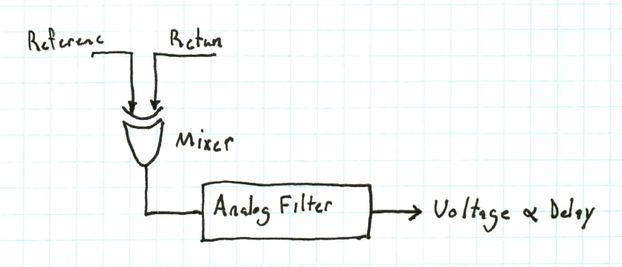This approach assumes that the transmitted pulse is periodic, as resolving a stable output voltage requires filtering over a large number of pulses.  The key to how this technique works is the mixer, which is simply analog-ese for 'multiplier'. If the signals are digital ones (as shown in this example), the mixer function can be implemented with an XOR (exclusive-or) gate.  The way in which this works is if there is no delay, the reference and return are identical, or 'in-phase', so when you XOR the two signals, you get a constant LOW (0V) output. If the delay is equal to half the period, the two signals are completely out-of-phase, which yields a constant HIGH (5V) output.  At delays between these extremes, the XOR gate's output is a duty-cycle proportional to the delay (Figure 8).  When you apply a low-pass filter function to this signal, you end up with a voltage that is proportional to the time delay. This voltage can then be measured with an analog-to-digital converter (ADC) to very high degrees of precision.  For example, if you were to pulse the reference with a 1us period, and use a 16-bit ADC to measure the output voltage, you could theoretically resolve the delay down to about 8 picoseconds.

Figure 8 - Phase Measurement Signals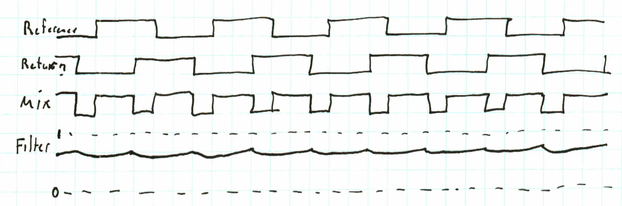One important measurement issue associated with the phase-based time measurement described above is that phase 'wraps around' - meaning that while phase delay may go from 0 degrees out to infinity, it is only uniquely measurable over a 0-180 degree range. For the example above, with a 1us pulse frequency, as one increases the delay from 0 to 500ns, the measured phase will increase from 0 to 180 degrees of the referecne waveform. If delay is increased further, past 500ns, then the measured phase will actually decrease until it reaches a minimum of 0 degrees at 1us. And this cycle will repeat for every additonal microsecond of delay added.  One way of getting aorund this problem is combining measurement techniques, for example phase meaurement with a digital counter. In this scenario the digital counter keeps track of the 'coarse' part of the measurement while the phase measurement is used for the 'fine' part of the measurement.

One of the most exciting class of up-and-coming applications, however, for TOF ranging is time is 3D depth sensing, where the depth of each partion of a scene is measured. To do this requires an array of pixels, as in a digital camera, except instead of reporting light intensity and color, the camera reads out the distance of each pixel in the scene. An example of this type of 3D camera is the Texas Instruments OPT8241 with 320 x 240 pixels.

All of the above examples have assumed that the return signal has been nicely amplified and thresholded so that it is represented by a nice clean digital pulse. While this may not be an unrealistic technique for a  ranging instrument, it is relatively expensive in terms of circuitry.  Also, an optical ranger may only be looking at a spot projected by a high-power laser, which represents a relatively easy signal to discriminate.  In a 3D camera, however, an entire scene needs to be illuminated, and the amount of light received by each pixel may vary.  Also, to maintain bandwidth for an imaging array with potentially tens or hundreds of thousands of individual pixels, it may be necessary to provide parallel delay-measurement chains on a single imaging chip.

This white paper from Texas Instruments describes a simple analog time-measurement technique that can handle variable amplitude return signals, as opposed to discriminated and cleaned-up digital signals.  The essence of this method is to provide two timing 'windows' which direct the return signal to be integrated (accumulated) into two separate buckets.  Figure 9 shows a conceptual implementation. When window 1 (W1) is active, the current from the receiver photodiode is integrated onto capacitor C1, resulting a voltage proportional to product of the photodiode current  and the time interval the window is active (total charge). Similarly, when window 2 (W2 ) is active, the signal is integrated by capacitor C2.

Figure 9 - Window-based Measurement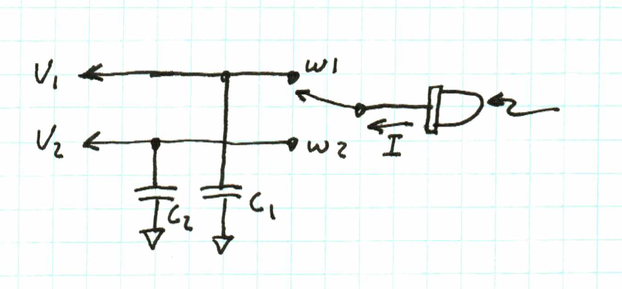The two windows are timed so that window W1 is in phase with the transmitted pulse, and window W2 comes immediately afterwards, with no overlap or gap between the two windows (Figure 10). After a W1/W2 cycle, the two capacitors C1 and C2 are charged to voltages V1 and V2, which can then be read by an ADC.

Figure 10 - Window-based Signals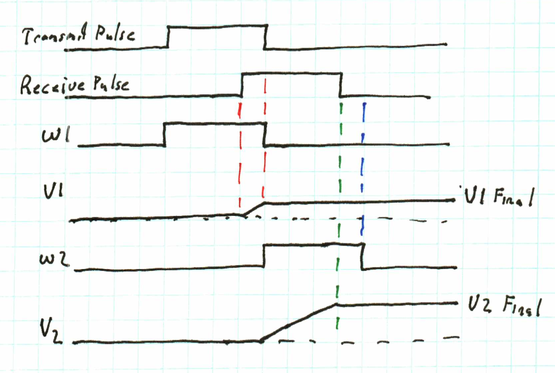The beauty of this technique is that the total amount of light received is represented by the sum of the voltages V1 and V2, and the delay can be calculated as V2/(V1+V2).  This means that the delay measurement is independent of the total amount of signal received by the photodiode.  This is particularly important in a camera-type device as it is normally expected that individual pixels will not receive the same amount of light back - after all, the difference in pixel values is what defines an image.  Another neat feature of this technique is that the necessary circuitry can be conceptually quite simple. While it might not be simple enough to provide every pixel with its own timing measurement unit, it might be possible to provide one for each row of pixels. This would make it possible to maintain decently fast frame rates even with a very high resolution array.

For a technology that has been around for several decades, optical TOF ranging just gets more and more interesting - especially when applied to imaging arrrays!